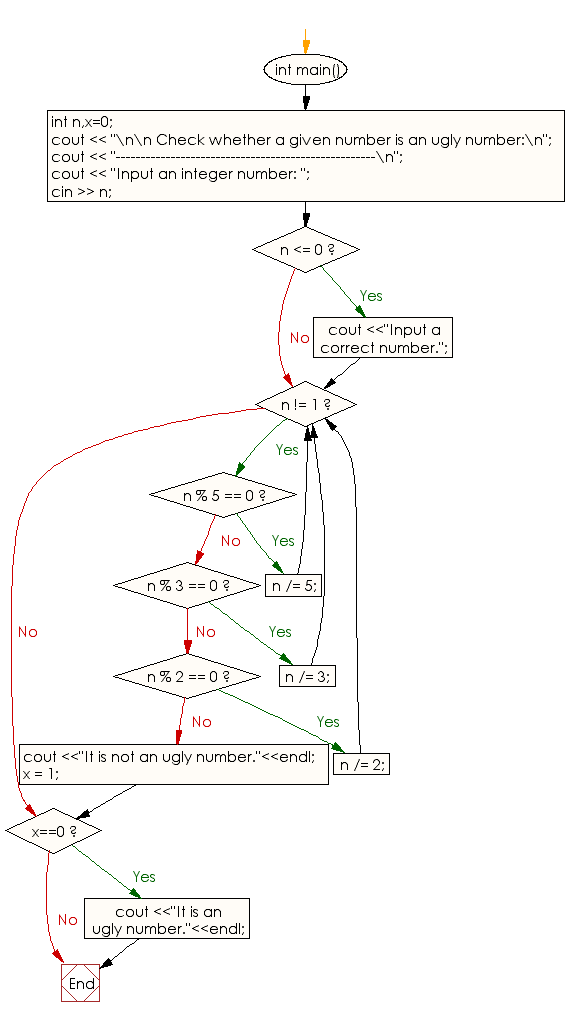﻿ C++ : Check whether a given number is an Ugly number or not

# C++ Exercises: Check whether a given number is an Ugly number or not

## C++ Numbers: Exercise-1 with Solution

Write a program in C++ to check whether a given number is an Ugly number or not.

Pictorial Presentation:Sample Solution:

C++ Code :

``````# include <iostream>
# include <string>
using namespace std;
int main()
{
int n,x=0;
cout << "\n\n Check whether a given number is an Ugly number:\n";
cout << "----------------------------------------------------\n";
cout << "Input an integer number: ";
cin >> n;
if (n <= 0) {
cout <<"Input a correct number.";
}
while (n != 1)
{
if (n % 5 == 0)
{
n /= 5;
}
else if (n % 3 == 0)
{
n /= 3;
}
else if (n % 2 == 0)
{
n /= 2;
}
else
{
cout <<"It is not an Ugly number."<<endl;
x = 1;
break;
}
}
if (x==0)
{
cout <<"It is an Ugly number."<<endl;
}
}
``````

Sample Output:

```Check whether a given number is an Ugly number:
----------------------------------------------------
Input an integer number: 25
It is an Ugly number.
```

Flowchart:C++ Code Editor: